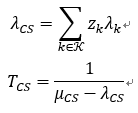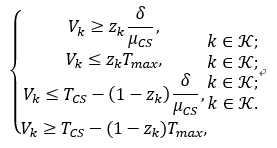# How to solve this problem? Cannot perform the operation: {real affine} <= {convex}

Hey guys,

As part of my objective function, a queuing delay formula is known as follows.where z is a binary decision variable. lambda ,mu and delta are pre-defined positive constants.
I used lambda_CS = sum(z .* lambda_k) and T_CS = inv_pos(mu_CS - lambda_CS) to represent the above two variables.
In my objective function, need to calculate z .* T_CS. But I receive the consequence of

Disciplined convex programming error:
Cannot perform the operation: {real affine} .* {convex}

So I use the Reformulation Linearization Technique (RLT) to transform the variables as follows,

cvx_begin
variable z(K) binary
variable V(K) nonnegative
expression lambda_CS
expression T_CS
lambda_CS = sum(z .* lambda_mean);
T_CS = inv_pos(mu_CS - lambda_CS);
minimize(sum(V));
subject to
lambda_CS <= (mu_CS - 1);
for k = 1:K
V(k) >= (z(k) / mu_CS );
V(k) <= (z(k) * T_max);
V(k) <= (T_CS - 1 / mu_CS + z(k) / mu_CS );
V(k) >= (T_CS - T_max + z(k) * T_max);
end
cvx_end

where T_max is a large positive constants.
I hope that through these four unlimited constraints, the equivalence is established. However, when I put these four unlimited constraints into the subject to, I receive the consequence of

Disciplined convex programming error:
Invalid constraint: {real affine} <= {convex}

The reason is that there is a problem with the third constraint.
What should I do? Looking forward to the help. It‘d be much appreciated if anyone could help me out. Thanks a lot.

This

Invalid constraint: {real affine} <= {convex}

tells you that your model is not convex as formulated. If you have

f(x) <= g(x)

then f(x) must be convex (e.g. affine) and g(x) must be concave. You violate this rule.

Hello Sir, thanks a lot for taking time to review my problem.
In my objective function, z is a binary variable and T_CS is a convex function. I can’t multiply the two, i.e. z .* T_CS. This gives: Cannot perform the operation: {real affine} .* {convex}.
So I define an auxiliary variable V and want to make V = z .* T_CS constant with the following four inequality constraints.When z_k = 0, the third and fourth constraints hold obviously and we can get 0 ≤ V_k ≤ 0 from the first and second constraint, which implies V_k= 0. When z_k = 1, the first and second constraints hold obviously and we have T_CS <= V_k <= T_CS from the third and fourth constraints, which indicates V_k = T_CS. As a result, we can obtain V_k = z_k * T_CS.
V is just an auxiliary variable, but T_CS is a convex function, so the program reports an error: {real affine} .* {convex}.
But I have no idea to make z .* T_CS. Is there any solution please?

Somewhat related CVX Expressions for Erlang Delay Formula